# Using If-Else Statements in Pandas: A Practical Guide [+ Examples]Updated:

Published:

One of the core benefits of programming is automation. Instead of doing something manually, you issue instructions to a computer that executes the task for you.To ensure the program returns the expected result, you need to provide explicit guide rails that instruct the computer how to respond in various scenarios. In programming, this concept is known as control flow. A major piece of control flow is defining computer logic, and one of the fundamental methods for providing this framework for a program is the if-else statement.

pandas is a Python library built to work with relational data at scale. As you work with values captured in pandas Series and DataFrames, you can use if-else statements and their logical structure to categorize and manipulate your data to reveal new insights.

Let's break down how to use if-else statements in pandas, starting with how to define the statements themselves.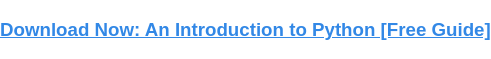## Pandas If Else Statement

Before diving into how to use if-else statements in pandas, let's break down the basic syntax.

In this example, you have a Series of test scores and want to know how many values are above the passing benchmark. You can inspect the Series below.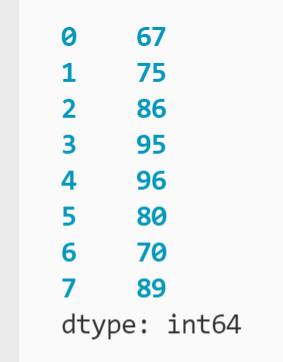To start understanding your data, you can implement a for loop to look at each value in your Series:

``` ```
pass_count = 0

pass_count += 1
``````

Let's break drown each level of this statement:

• pass_count = 0: A variable to hold the results of the for loop with a placeholder value of 0.
• if grade >= 70: An if statement that evaluates if each grade is greater than or equal to (>=) the passing benchmark you define (70).
• pass_count += 1: If the logical statement evaluates to true, then 1 is added to the current count held in pass_count (also known as incrementing).

This loop will continue until each number in grade_series has been evaluated. You can then print the results to the terminal:

``` ```
print("Number of passing tests:", pass_count)
``````

The output is below.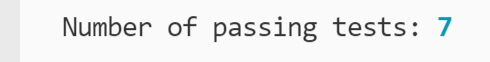If you wanted to know the inverse of the pass count — how many tests failed — you can easily add to your existing if statement:

``` ```
pass_count = 0
fail_count = 0

pass_count += 1
else:
fail_count += 1
``````

Here, else serves as a catch-all if the if statement returns false. In other words, the statement tells the program if the grade is greater than or equal to 70, increase pass_count by 1 — otherwise, increase fail_count by 1. No matter the actual score value, if it doesn't meet the condition in the if statement, the code defined beneath else is executed.

The results of the new if-else statement are below.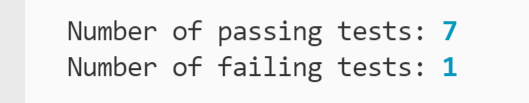This logic works because you have a binary condition. Either the test passes or it doesn't. However, not every scenario will have only two outcomes. In these cases, you can broaden the conditions evaluated with the elif (short for "else if") statement.

Now, say you want to take the numerical test scores and find their letter grade equivalents. Like before, you are interested in only aggregate counts versus the individual scores.

In this example, you define a dictionary to hold the counts of each grade as a property under one variable: letter_grades. You then update the for loop with elif statements:

``` ```
'a_count': 0,
'b_count': 0,
'c_count': 0,
'd_count': 0,
'f_count': 0
}

else:
``````

You are still using an if statement at the start and an else statement at the end. But now you have three elif statements between them to account for additional outcomes. A test score could evaluate as an A, B, C, D, or F, so the binary if-else statement from the last example would not suffice.

The for loop will move through each statement and stop at the first condition that evaluates to true. It will not execute any following statements after the first true condition, so a grade that evaluates to an A will not also increase the count of the other properties. If none of the conditions return true, it executes the else statement.

The outcome of executing the expanded for loop is below.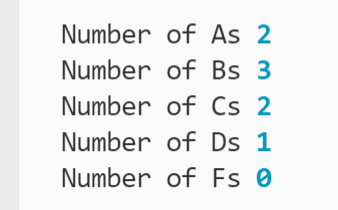You have now seen some use cases for if-else statements in pandas. However, imagine if you wanted to share the letter count or pass/fail data elsewhere. You would need to remember to export not only the original Series but any aggregated variables (e.g. letter_grades). This can easily become overwhelming if you are performing multiple calculations as part of your analysis.

It is common practice to store the results of evaluations in a new column. This would convert a Series into a DataFrame or simply expand an existing DataFrame. Let's examine how to use if-else statements with DataFrames next.

## How to Use If Else Statements in a Pandas DataFrame

### 1. The .apply Method

Best for: applying a custom function with a traditional if-else statement to evaluate columns and store the results

One of the simplest ways to apply if-else statements to a DataFrame is with the .apply method. In short, .apply applies a function you define to each full row or row subset of a DataFrame. You can define your function to return a result based on conditional statements.

In this example, you have a DataFrame holding student names and their corresponding test scores: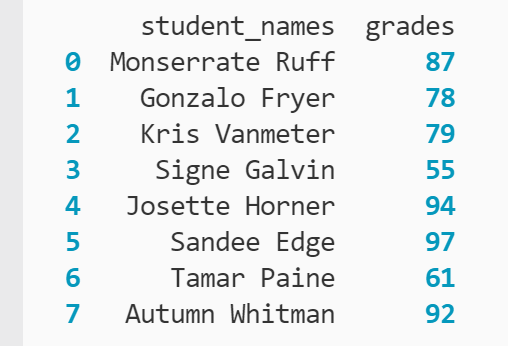Borrowing the logic defined in the last example, you can apply a custom function that returns the letter grade that corresponds to each numerical test score by calling .apply():

``` ```
def assign_letter(row):
if row >= 90:
result = 'a'
elif row >= 80:
result = 'b'
elif row >= 70:
result = 'c'
elif row >= 60:
result = 'd'
else:
result = 'f'
return result

``````

First, you declare a function with the def keyword and assign the function a name (assign_letter) so you can pass it as an argument in .apply(). assign_letter() takes one argument (row), which is a placeholder for the values that will be passed in for each row in the DataFrame.

Within assign_letter(), you have an if-else statement that evaluates the row values. Whenever a condition is met, the temporary variable result is declared that stores the letter grade as a string. Since the if-else statement stops execution once one statement evaluates to true or else is reached, result is immediately returned, and .apply() moves to the next row.

.apply() runs the assign_letter() function against each row and compiles a Series of the results. In this case, the indexing operator ([ ]) is used to specify that .apply() only targets the values contained under the "grades" column versus the full rows of the grades_df DataFrame. Otherwise, assign_letter() will attempt to evaluate whether the student name strings are greater than or equal to the integers you provided, resulting in a TypeError.

The result of calling .apply is below.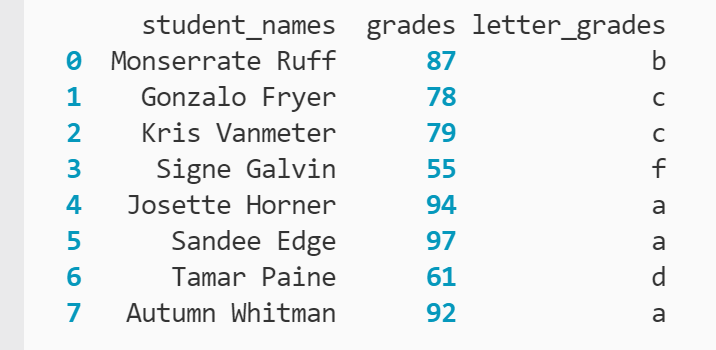The .apply method works well for multi-conditional scenarios like assigning multiple letter grades. If the evaluation is binary, however, you can simplify the workflow with .loc.

### 2. The .loc Method

Best for: quickly defining simple logical statements in a few lines

The .loc method is an indexing operator that you can use to quickly evaluate row values when the condition has a binary outcome (either it is true or it is false).

For this example, the DataFrame holds numerical test scores for students, and you want to evaluate whose tests passed.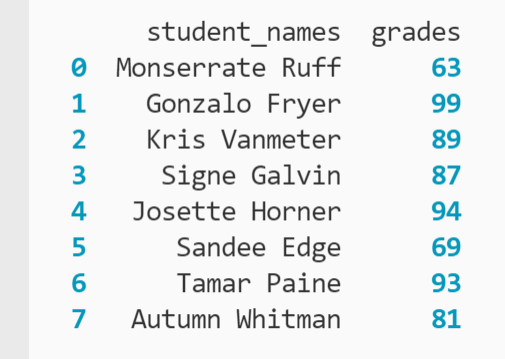To start, you invert the control flow of the if else statement by assigning the catch-all (else) value first:

``` ```
``````

Here, you have created a new column named "passing" and assigned it a universal value of the boolean False. You can see the result below.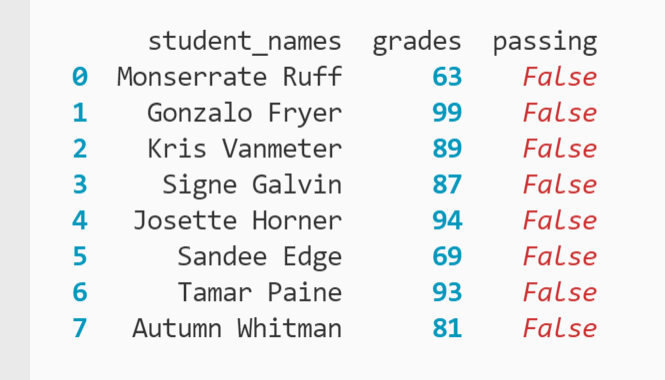However, you know it's not likely that every student failed the test (hopefully). To confirm this, you can now assign the passing condition:

``` ```
``````

.loc[] is used to look for values under the "grades" column where the value is greater than or equal to 70. It then assigns the boolean True to the cell under the "passing" column of the corresponding row, overwriting the existing False. The output is printed below.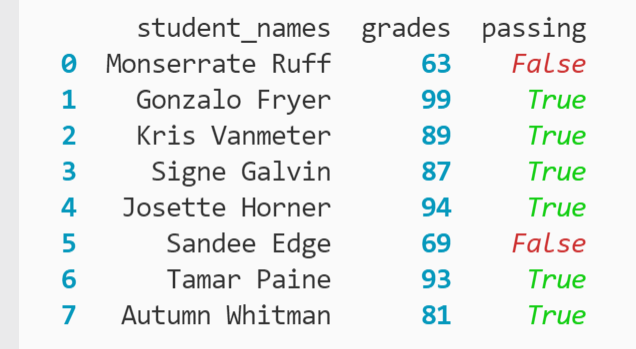After the evaluations finish executing, you can see that you have six passing tests and only two failing tests. You could define more than one condition with .loc, but it can quickly become unwieldy to track them in separate statements.

Now that you're more familiar with if-else statements, let's look at another method for defining multiple logical statements: the Numpy .select method.

### 3. The NumPy .select Method

Best for: evaluating multiple conditions with the most efficient turnaround time of any method

Like the .apply method, .select allows you to define multiple conditions to evaluate the DataFrame. However, .select is not native to Python. Instead, you need to import the NumPy library before calling it:

``` ```
import pandas as pd
import numpy as np
``````

Now that you've added your NumPy import statement beside the existing pandas import, you're ready to start using .select.

This example will return to the use case of assigning letter grades based on test scores. The base DataFrame is below.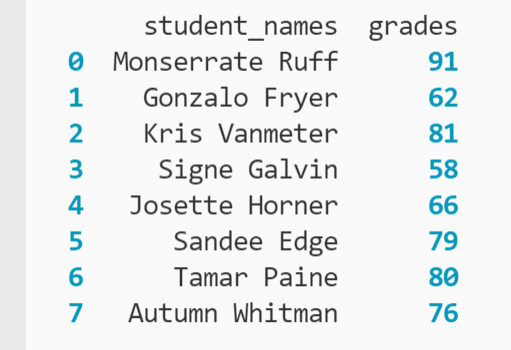The first step is to define your conditions:

``` ```
conditions = [
]
``````

Here, you are declaring a variable conditions that holds a list. Each condition is separated by a comma. Note the ampersand (&) joining different comparison operators, which declares that a value must meet both conditions specified. For example, a value in the "grades" column must be greater than or equal (>=) to 60 and less than (<) 70.

Next, you declare another list to hold the values each condition will correspond to, in this case the letter grade strings:

``` ```
letters = ['f', 'd', 'c', 'b', 'a']
``````

Note that you need to match the order of the values to the order of conditions. Otherwise, scores below 60 would be marked as "a" and so on.

Now that you have declared both arguments, you're ready to call .select():

``` ```
``````

Here, you are creating a new column with the label "letter_grades" and setting it equal to the result of calling .select() from the NumPy (np) library. The method takes the conditions and letters lists as arguments and returns a list of results based on evaluating each row under the "grades" column.

You can confirm .select performed as expected by printing the DataFrame to the terminal: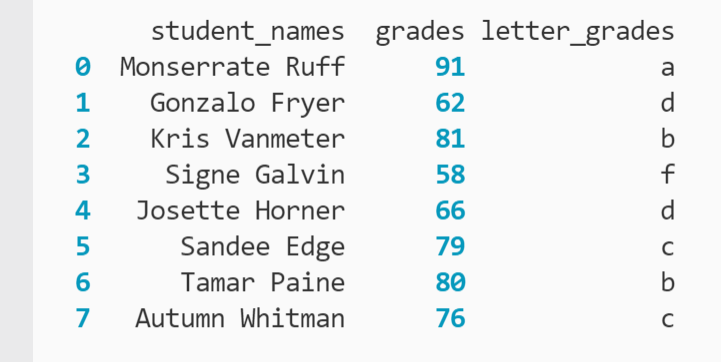The combined code is below.

``` ```
conditions = [
]

letters = ['f', 'd', 'c', 'b', 'a']

``````

This method requires an additional library and has more lines than the .apply method, so you may wonder why it's useful when there's already a method for evaluating multiple conditions. Where .select outshines .apply is execution speed.

On a small DataFrame like these examples have used, the turnaround time between each of these methods is negligible. However, increase the DataFrame size to thousands or tens of thousands of rows, and efficient methods are crucial to finding answers quickly.

This video from datagy gives a live demo of the three methods we've reviewed so far and even walks through an advanced use case for mapping a Python dictionary's values to a DataFrame:

## Use if-else statements in Pandas to find the answer faster.

If-else statements are a fundamental component of control flow in programming. When it comes to data analysis in pandas, they offer a convenient way to segment the data and produce new insights. Python, combined with its pandas and NumPy libraries, offers several strategies to incorporate if-else statements and their underlying logic into your analysis to better understand your data and apply it to your most pressing business challenges.Topics: What Is Python?

## Related Articles

We're committed to your privacy. HubSpot uses the information you provide to us to contact you about our relevant content, products, and services. You may unsubscribe from these communications at any time. For more information, check out our Privacy Policy.

A guide for marketers, developers, and data analysts.